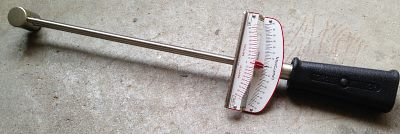calculator.org

property>torque

What Is Torque?The torque around an axis of rotation is a measure of the turning force on an object connected to that axis. Many people think of this more simply as the intensity of a twisting force. For a given lever arm connected to an object to be turned (think of a wrench and a bolt), the torque on that bolt will depend on the length of the lever arm, where one is pushing on the lever arm, and the magnitude of the force. Torque is symbolized by the Greek letter τ and has SI units of newton meters. A special tool called a torque wrench can be used to set a specified maximum amount of torque to apply to tighten a bolt - when the set torque is exceeded the wrench will allow the bolt to slip.

Torque is related to angular momentum, angular acceleration, and angular velocity in the following way: if we assume there is a particle p, and a lever arm of length r, then we will find that the torque on our particle is equal to the cross product of a force F applied perpendicular to the lever arm at the distance r. In other words:

τ = r x F

τ = r.F.sin(θ)

The angle θ is given by the angle between the force vector F and the position vector r. F also has a component that runs perpendicular to r, and this component alone can also be used to calculate the magnitude of the torque.

τ = r.Fperpendicular

The torque on a body is also the rate of change of the angular momentum of that body. In other words:

τ = dL/dt

and

L = I.ω

In the second equation, we are relating the angular momentum to the moment of inertia, I, and angular velocity ω. We can go even further and differentiate both sides of the equations to get the following:

dL/dt = d(Iω)/dt = I.(dω/dt) = I.α

Here, dω/dt is taken to be the rate of change of angular velocity, which is also known as angular acceleration, or α. We end up going coming full circle and substituting I*α for dL/dt, to relate torque to the moment of inertia I and α to get:

τ = I.α

This equation is very similar to the classic equation F = ma insofar as torque is the angular analogue of force and the moment of inertia is the angular analogue of mass (often referred to as angular mass).

Bookmark this page in your browser using Ctrl and d or using one of these services: (opens in new window)